# Bihar Board Class 10 Science Solutions Chapter 5 Periodic Classification of Elements

## BSEB Bihar Board Class 10 Science Solutions Chapter 5 Periodic Classification of Elements

Bihar Board Class 10 Science Solutions Chapter 5 Periodic Classification of Elements Textbook Questions and Answers, Additional Important Questions, Notes.

### Bihar Board Class 10 Science Chapter 5 Periodic Classification of Elements InText Questions and Answers

Intext Questions (Page 81)

Question 1.
Did Dobereiner’s triads also exist in the columns of Newlands’ Octaves ? Compare and find out.
Yes. Dobereiner’s triads also exist in the columns of Newlands’ Octaves. For example, Li, Na, K is a triad of Dobereiner which also exist in the columns of Newlands’ Octaves.

Question 2.
What were the limitations of Dobereiner’s classification ?

• Dobereiner could not classify all the elements known at that time.
• Dobereiner could identify only three triads from the elements known at that time.
• So the system of classification into triads was not accepted.

Question 3.
What were the limitations of Newlands’ Law of Octaves?

• Newlands’ Law of Octaves was applicable only upto calcium. After calcium the eighth element did not possess properties similar to that of the first.
• Newlands assume that only 56 elements existed in nature and no more elements would be discovered in the future.
• Newlands’ Octaves contain only few elements having similar properties.

Intext Questions (Page 85)

Question 1.
Use Mendeleev’s Periodic Table to predict the formulae fgr the oxides of the following elements :
K, C, Al, Si, Ba
K → K2O
C → CO2
Al →Al2O3
Si → SiO2
Ba →BaO

Question 2.
Besides gallium, which other elements have since been discovered that were left by Mendeleev in his periodic table ? (any two)
(i) Scandium
(ii) Germanium.Question 3.
What were the criteria used by Mendeleev in [ creating his Periodic Table ?
(i) He arranged the elements on the bases of their increasing, atomic masses.
(ii) Second criteria was the similarity in the chemical properties.
(iii) The formulae of hydides and oxides formed by an element were treated as one of the basic property of an element for the classification used by Mendeleev.

Question 4.
Why do you think the noble gases are placed in a separate group ?
Noble gases are places in separate group because :

• These gases discovered very late as they are very inert.
• These gases could be placed in a new group without : disturbing the existing order.

Intext Questions (Page 90)

Question 1.
How could the Modern Periodic Table remove various anomalies of Mendeleev’s Periodic Table?

• In Modern Periodic Table, the place of hydrogen is justified because it is electropositive and so it is placed in first group with metals.
• In Modern Periodic Table, the elements are arranged on the basis of atomic number. So the place of isotopes is also justified as the atomic number remain same of all the isotopes.
• The order of heavy and light elements is also corrected in the Modern Periodic Table.
• The position of inert gases is also justified.

Question 2.
Name two elements you would expect to show chemical reactions similar to magnesium. What is the basis for your choice ?
Calcium (Ca) and Barium (Ba). Basis of choice of these two elements :

• These two elements belong to same group as magnesium.
• These two have the same valence electrons as magnesium has

Question 3.
Name :
(a) three elements that have a single electron in their outermost shell.
(b) two elements that have two electrons in their outermost shells.
(c) three elements with filled outermost shells.
(a) Lithium (Li) Sodium (Na), Potassium (K).
(b) Magnesium (Mg), Calcium (Ca), Barium (Ba).
(c) Helium (He), Neon (Ne), Argon (Ar).Question 4.
(a) Lithium, sodium and potassium are all metals that react with water to liberate hydrogen gas. Is their any similarity in the atoms of these elements ?
(b) Helium is an unreactive gas and neon is a gas of extremely low reactivity. What if anything do their atoms have in common ?
(a) Lithium, sodium and potassium atoms have same number of electrons in their outermost shell and have same valency.
(b) Helium and neon both have their outermost shell filled.

Question 5.
In the Modern Periodic Table, which are the metals among the first ten elements ?
Lithium, beryllium, boron are the metals in Modern Periodic Table among the first tfen elements.

Question 6.
By considering their positions in the periodic table, whic’* one of the following elements would you expect to have maximum metallic characteristics ? Ga, Gre, As, Se, Be
Be has the maximum metallic characteristics because all other elements are situated at the right hand side in periodic table than Be. Due to the position their metallic characteristics decrease as we go from left to right.

### Bihar Board Class 10 Science Chapter 5 Periodic Classification of Elements Textbook Questions and Answers

Question 1.
Which of the following statements is not a correct statement about the trends when going from left to right across the periodic table.
(a) The elements become less metallic in nature.
(b) The number of valence electrons increases.
(c) The atoms lose their electrons more easily.
(d) The oxides become more acidic.
(c) The atoms lose their electrons more easily.

Question 2.
Element X forms a chloride with the formula XCl2 which is a solid with a high melting point. X would most likely be in same group of the periodic table as :
(a) Na
(b) Mg
(c) Al
(d) Si
(e) P
(b) Mg, because the chlorides of this group are XCl2.

Question 3.
Which element has
(a) two shells, both of which are completely filled with electrons.
(b) the electronic configuration 2,8, 2.
(c) a total of three shells with four electrons in its valency shell.
(d) a total of two shells with three electrons in its valency shell.
(e) twice as many electrons in its second shell as in its first.
(a) Neon (Ne)
(b) Magnesium (Mg)
(c) Silicon (Si)
(d) Boron (B)
(e) Carbon (C)Question 4.
(a) What property do all elements in the same column of the periodic table as boron have in common ?
(b) What property do all elements in the same column of the periodic? table as fluorine have in common?
(a) Both the elements are metal and show the following common properties:

• Both are good conductor of electricity.
• Both show malleability.

(b) Both the elements are non-me,tal and show following common properties : ‘

• Both are brittle.
• Both are bad conductor of electricity.

Question 5.
An atom has electronic configuration 2, 8, 7.
(a) What is the atomic number of,this element?
(b) To which of the following element would it be chemically similar (Atomic numbers are given in parentheses)
N (7), F (9), P (15), Ar (18)
(a) The atomic number of element is 17.
(b) It belongs chemically to F (9) because the electronic
configuration of F is 2, 7 and having same Valence electrons.

Question 6.
The position of three elements A, B and C in the periodic table are shown below :(a) State whether C is metal or non-metal?
(b) State whether C is more reactive or less reactive than A?
(c) Will C be larger or smaller than B ?
(d) Which type of ion, cation or anion, will be formed by element A ?
(a) C is non-metal because it belongs to 17th group.
(b) C is less reactive than A because the reactivity of non-metal decrease from top to bottom.
(c) The size of C is smaller than B because B and C belong to the same period and the size is decrease in a period on going left to right.
(d) A forms anion because C is non-metal and non-metals for anion (Negative Ion).

Question 7.
Nitrogen (atomic number 7) and phosphorus (atomic number 15) belong to group 15 of periodic table. Write the electronic configuration of these two elements. Which of these will be more electronegative ? Why ?
Atomic number of nitrogen is 7
Electronic configuration : 2, 5
Atomic number of phosphorus is 15
Electronic configuration : 2, 8, 5
Phosphorus will be more electronegative because phosphorus and nitrogen both are non-metals. Phosphorus is situated in the lower side than nitrogen. In non-metals, as we go top to bottom the electronegativity is increased.Question 8.
How does the electronic configuration of an atom relate to its position in the Modern Periodic Table ?
The electronic configuration is related to the position of elements in periodic table. The number of electrons in outermost . shell show the number of group and the number of shells show [ the number of periods. »

Question 9.
In the Modern Periodic Table, calcium (atomic number 20) is surrounded by elements with atomic numbers 12, 19, 21, 28. Which of these have physical and chemical properties resembling calcium ?
Calcium atomic number is 20.
Electronic configuration is 2, 8, 8, 2.
Calcium surrounded by elements.

1. Atomic number – 12
Configuration – 2, 8, 2

2. Atomic number – 19
Configuration – 2, 8, 8, 1

3. Atomic number – 21
Configuration – 2, 8, 8, 3

4. Atomic number – 38
Configuration -: 2, 8, 18, 8, 2
So, the elements having atomic number 12 and 38 resemble to the properties of calcium.

Question 10.
Compare and contrast the arrangements of elements in Mendeleev’s Periodic Table and the Modern Periodic Table.

• Mendeleev’s Periodic Table is based on atomic mass while Modern Periodic Table is based on atomic number.
• In Mendeleev’s table there is no place of inert gases while in Modern Periodic Table they are classified.
• In Modern Periodic Table all the anomalies of Mendeleev’s Periodic Table are removed.
• There are 8 groups in Mendeleev’s Periodic Table while there are 18 groups in Modern Periodic Table.

### Bihar Board Class 10 Science Chapter 5 Periodic Classification of Elements Textbook Activities

Activity 5.1 (Page 84)

Question 1.
Looking at the resembleness to alkali metals and the halogen family, try to assign hydrogen a correct position in Mendeleev’s Periodic Table.
Mendeleev placed hydrogen with alkali metals while its some properties, are similar to halogens. The position of hydrogen in Mendeleev’s Periodic Table is correct because its properties are more similar to the, alkali metals as it has the property of losening the electrons, i.e., it is electropositive.

Question 2.
To which group and period should hydrogen be assigned ?
First group and first period.Activity 5.2 (Page 85)

Consider the isotopes of chlorine, Cl – 35 and Cl – 37.

Question 1.
Would you place them in different slots because their atomic masses are different ?
No, because the more fundamental base of classification is atomic number and not atomic mass.

Question 2.
Or would you place them in the same position because their chemical properties are the same ?
Yes, both the isotopes are placed in same position because they have same chemical properties, and same atomic number.

Activity 5.3 (Page 85)

Question 1.
How were the positions of cobalt and nickel resolved in the Modern Periodic Table ?
Modern Periodic Table is based on the atomic number.’ r ‘ Cobalt (27) is placed before Nickel (28) in Modern Periodic Table.

Question 2.
How were the positions of isotopes of various y elements decided in Modem Periodic Table ?
The isotopes have same atomic number so they are placed in the same group in Modern Periodic Table.

Question 3.
Is it possible to have an element with atomic number 1.5 placed between hydrogen and helium ?
No, it is not possible because atomic number is a whole number.

Question 4.
Where do you think should hydrogen be placed in Modem Periodic Table ?
I think that the place of hydrogen in the Modem Periodic Table is correct.

Activity 5.4 (Page 87)

Question 1.
Look at the group 1 of Modem Periodic Table and name the elements present in it.
The elements in group 1 are :
Hydrogen (H)
Lithiuih (Li)
Sodium (Na)
Potassium (K)
Rubidium (Rb)
Caesium (Cs)
Francium (Fr)

Question 2.
Write down the electronic configuration of the first three elements of group I.
(i) H (1) → 1
(ii) Li (3) → 2, 1 N .
(iii) Na (11) → 2, 8, 1.

Question 3.
What similarity do you find in their electronic configuration ?
All the elements have same number of valence electron, i.e., 1.

Question 4.
How many valence electrons are present in these three elements ?
One (1).Activity 5.5 (Page 87)

Question 1.
If you look at the long form of the Periodic Table, you will find that the elements, Li, Be, B, C, N, O, F and Ne are present in the second period. Write down their electronic configuration.
Li (3) → 2, 1
Be (4) → 2, 2
B (5) → 2, 3
C (6) → 2, 4
N (7) → 2, 5
O (8) → 2, 6
F (9) → 2, 7
Ne (10) → 2, 8

Question 2.
Do these elements also contain the some number of valence electrons?
No.

Question 3.
Do they contain the seme number of shells ?
Yes.
Note : The elements of some periods have same number of shells but riot Same number of valence electrons (It increases by 1)

Activity 5.6 (Page 88)

Question 1.
How do you calculate the valency of an element from its electronic configuration ?
Valency of metals : It is same as the number of valence electrons, i.e., 1, 2 and 3.,
Valency of non-metals : Valency of non-metals can be calculated by (8 – valence electrons).
For example,
8 – 4 = 4,
8 – 5 = 3,
8 – 6 = 2
8 – 7 = 1
8 – 8 = 0

Question 2.
What is the valency of magnesium with atomic number 12 and sulphur with atomic number 16 ?
Magnesium (12) → 2, 8, 2
Sulphur (16) → 2, 8, 6
The valency of magnesium is same as valence electron, i.e. 2.
The valency of sulphur is 8 – 6 = 2 because it is a non-metal.

Question 3.
Similarly find out the valencies of the first 20 elements.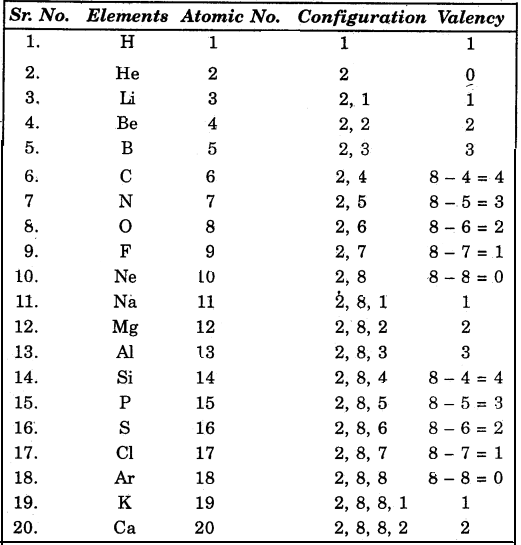Question 4.
How does the valency vary in period on going from left to right ?
Valency first increases 1 to 4 then decreases from 4 to 0 (1, 2, 3, 4, 3, 2, 1, 0).Question 5.
How does the valency vary in going down in a group ?
Valency remains the saipe because valence electrons do not change on going down in a group.

Activity 5.7 (Page 88)

Atomic radii of the elements of the second period are given as follows :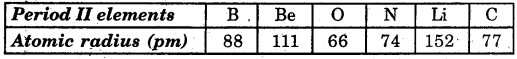Question 1.
Arrange them in decreasing order of their atomic radii.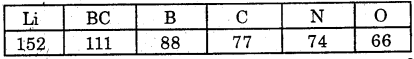Question 2.
Are the elements now arranged in the pattern of a period in the periodic table ?
Yes.

Question 3.
Which elements have the largest and smallest atoms ?
Largest atoms : Li Smallest atoms :

Question 4.
How does the atomic radius change as you go left to right in a period ?
The atomic radius decreases as we go left to right in a period.Activity 5.8 (Page 89)

Question 1.
Study the variations in the atomic radii of first group elements given below and arrange them in an increasing order.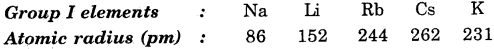Question 2.
Name the demerits which have the smallest and the largest atoms.
Smallest atom : Na (Sodium)
Largest atom : Ca (Calcium)

Question 3.
How does the atomic size vary as you go down ” a groupi ?
Atomic size is increased as we go down a group.

Activity 5.9 (Page 89)

Question 1.
Examine the elements of the third period and classify them as metals and non-metals.
Elements of period III : Na (11), Mg (12), A1 (13), Si (14). P (15). S (16). Cl (17). Ar (18)Note : The elements having 1,2,3 valence electrons are metals while the elements having 4,5,6, 7,8 valence electrons are non-metals.

Question 2.
On which side of the periodic table do you find the metals ?
On the left side.

Question 3.
On which side of the periodic table do you find the non-metals?
On the right side.Activity 5.10 (Page 89)

Question 1.
How do you think the tendency to lose electrons will, change in a group ?
The tendency of lose electrons increases in a group on going down.

Question 2.
How will this tendency change in a period ?
The tendency of lose electrons decreases as we go left to right in a period.

Activity 5.11 (Page 90)

Question 1.
How would the tendency to gain electrons change as you go from left to right across a period?
The tendency of gaining electrons increases as we go left to right in a period upto 17th group. It decreases in 18th group. . . ‘

Question 2.
How would the tendency to gain electrons change as you go down a group ?
The tendency of gaining the electrons decreases as we go down a group.

### Bihar Board Class 10 Science Chapter 5 Periodic Classification of Elements NCERT Exemplar Problems

Question 1.
The three elements A, B and C with similar properties have atomic masses X, Y and Z respectively, t The mass of Y is approximately equal to the average ; mass of X and Z. What is such an arrangement of elements called as? Give one example of such a set of elements.
The arrangement of these elements is known as Dobereiner triad. Example, Lithium, Sodium and Potassium.

Question 2.
Elements have been arranged in the following v sequence on the basis of their increasing atomic masses. ‘
F, Na, Mg, Al, Si, P, S, Cl, Ar, K
(a) Pick two sets of elements which have similar properties.
(b) The given sequence represents which law of classification of elements?
(a) (i) F and Cl (ii) Na and K,
(b) Newland’s Law of Octaves

Question 3.
Can the following groups of elements be classified as Dobereiner’s triad ?
(а ) Na, Si, Cl
(b) Be, Mg, Ca
Atomic mass of Be 9; Na 23; Mg 24; Si 28; Cl 35; Ca 40. Explain by giving reason.
(a) No, because all these elements do not have similar properties although the atomic mass of silicon is average of atomic masses of sodium (Na) and chlorine (Cl).
(b) Yes, because they have similar properties and the mass of magnesium .(Mg) is roughly the averagd of the atomic mass of Be and Ca.Question 4.
In Mendeleev’s Periodic Table the elements were arranged in the increasing order of their atomic masses. However, cobalt with atomic mass of 58.93 amu was placed before nickel having an atomic mass of 58.71 amu. Give reason for the same.
In Mendeleev’s Periodic Table the elements were arranged in the increasing order of their atomic masses. However, cobalt with atomic mass of 58.93 amu was placed before nickel having an atomic mass of 58.71 amu. The sequence was inverted so that elements with similar properties could be grouped together. .

Question 5.
Hydrogen occupies a unique position in Modern Periodic Table”. Justify the statement.
Hydrogen could be placed either in group 1 or group 17 in the first period because it resembles alkali metals as well as halogens. In the Modern Periodic Table, hydrogen is placed at the top of the 1st column. i.e., it is the 1st member of the 1st period. The place of hydrogen is justified because it is electropositive and so it is placed in first group with metals.

Question 6.
Write the formulae of chlorides of Eka-silicon and Eka-aluminium, the elements predicted by Mendeleev.
The formula of chloride of Eka-silicon is ECl2 and that of Eka-aluminium is ECl3 which were later found as GaCl3 and GaCl3 respectively.

Question 7.
Three elements A, B and C have 3, 4 and 2 electrons respectively in their outermost shell. Give the group number to which they belong in the Modern Periodic Table. Also, give their valencies.Question 8.
If an element X is placed in group 14, what will be the formula and the nature of bonding of its chloride?
If an element %’ is placed in group 14, its valency would be 4. So, the formula of its chloride would be XCl4 and would be covalent bonding in its chloride.

Question 9.
(а) X has 12 protons and 12 electrons
(b) Y has 12 protons and 10 electrons
The  species *X’ contains 3 shells while the species Y’ which is the cation of the species “X’ contains 2 shells. So, the radius of the species Y would be less than the radius of the species ‘X’.Question 10.
Arrange the following elements in increasing order of their atomic radii.
(a) Li, Be, F, N (&) Cl, At, Br, I
(a) F < N < Be < Li
(b) Cl < Br < I < At

Question 11.
Identify and name the metals out of the following elements whose electronic configurations are given below.
(a) 2, 8, 2
(b) 2, 8, 1
(c) 2,8,7
(d) 2, 1 ;
(a) (b) and (d)
(a) Magnesium
(b) Sodium
(d) Lithium ‘

Question 12.
Write the formula of the product formed when the element A (atomic number 19) combines with the element B (atomic number 17). Draw its electronic dot structure. What is the nature of the bond formed?
The atomic number of the element A is 19, its electronic configuration is 2, 8, 8, 1 and the atomic number of the element B is 17, its electronic configuration is 2, 8, 7. Thus, element A is capable of giving l e~ and element B is capable of accepting 1e. So the formula of the product formed when the element A combines with the element B is AB. Its electronic dot structure is An ionic bond is formed when A combines with B.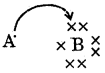From the above discussion it is clear that the element A is potassium (K) and the element B is chlorine (Cl). .

Question 13.
Arrange the following elements in the , increasing order of their metallic character
Mg, Ca, K, Ge, Ga
Ge < Ga < Mg < Ca < K

Question 14.
Identify the elements with the following property and arrange them in increasing order of their reactivity
(a) An element which is a soft and reactive metal
(b) The metal which is an important constituent of limestone
(c) The metal which exists in liquid state at room temperature.
(a) Na or K
(b) Ca
(c) Hg Hg < Ca < Na < K

Question 15.
Properties of the elements are given below. Where would you locate the following elements in the periodic table?
(a) A soft metal stored under kerosene
(b) An element with variable (more than one) valency stored under water
(c) An element which is tetravalent and forms the basis of organic chemistry
(d) An element which is an inert gas with atomic number 2
(e) An element whose thin oxide layer is used to make ‘ other elements corrosion resistant by the process of “anodising”
(a) Sodium (Na) Group 1 and Period 3 or Potassium (K) Group 1 and Period 4 .
(b) Phosphorus (P) Group 15 and Period 3 ~
(c) Carbon (C) Group 14 and Period 2
(d) Helium (He) Group 18 and Period 1
(e) Aluminium (Al) Group 13 and Period 3Question 16.
An element is placed in 2nd Group and 3rd Period of the Periodic Table, bums in presence of oxygen to form a basic oxide.
(a) Identify the element
(b) Write the electronic configuration
(c) Write the balanced equation when it burns in the presence of air
(d) Write a balanced equation when this oxide is dissolved in water
(e) Draw the electron dot structure for the formation of this oxide.
(a) The element which is placed in 2nd group and 3rd period of the periodic table, burns in presence of oxygen to form a basic oxide is magnesium (Mg).
(b) The electronic configuration of magnesium is(c) The balanced equation when it bums in the presence of air is as follows :
2Mg(s) + O2(g) → 2MgO(s)

(d) The balanced equation when this oxide is dissolved in water is as follows :
MgO(s) + H2O(l) → Mg(OH)2(aq)

(e) The electron dot structure for the formation of this oxide is given below :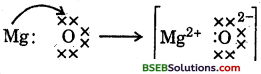Question 17.
An element X (atomic number 17) reacts with an element Y (atomic number 20) to form a divalent halide.
(a) Where in the periodic table are elements X and Y placed?
(b) Classify X and Y as metal(s), non-metal(s) or metalloid(s)
(c) What will be the nature of oxide of element Y? Identify the nature of bonding in the compound formed
(d) Draw the electron dot structure of the divalent halide.
(a) The element X (atomic number 17) lies in the Group 17 and 3rd period in the Modern Periodic Table and the element Y (atomic number 20) lies in the Group 2 and 4th period.
(b) The element X has a tendency to gain e~ so it is a non-metal and the element Y has a tendency to loose e so, it is a metal.
(c) As Y is a metal so its oxide would be basic in nature. In the divalent halide formed by the elements X and Y, the ionic bonds would be formed.
(d) The electron dot structure of the divalent halide is as follows :Question 18.
Atomic number of a few elements are given below 10, 20, 7, 14
(а) Identify the elements
(b) Identify the Group number of these elements in the Periodic Table
(c) Identify the Periods of these elements in the Periodic Table
(d) What would be the electronic configuration for each of these elements?
(e) Determine the valency of these elements.
(a) Elements – Neon (Ne), Calcium (Ca), Nitrogen (N), Silicon (Si)
(b) Group – 18, 2, 15, 14
(c) Period – 2, 4, 2, 3
(d) Electron configuration – (2, 8); (2,. 8, 8, 2); (2, 5); (2,8,4)
(e) Valency – 0, 2, 3, 4Question 19.
Complete the following cross word puzzle (Figure 5.1)
Across :
(1) An element with atomic number 12.
(3) Metal used in making cans and member of Group 14.
(4) A lustrous non-metal which has 7 electrons in its outermost shell.
Down:
(2) Highly reactive and soft metal which imparts yellow colour when subjected to flame and is kept in kerosene.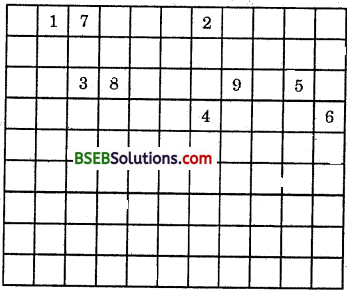(5) The first element of second Period
(6) An element which is used in making fluorescent bulbs and is second member of Group 18 in the Modern Periodic Table
(7) A radioactive element which is the last member of halogen family.
(8) Metal which is an important constituent of steel and forms rust when exposed to moist air.
(9) The first metalloid in Modern Periodic Table whose fibres are used in making bullet-proof vests.Question 20.
(a) In this ladder (Figure 5.2) symbols of elements are jumbled up. Rearrange these symbols of elements in the increasing order of their atomic number in the Periodic Table.
(b) Arrange them in the order of their group also.(a) H, He, Li, Be, B, C, N, O, F, Ne, Na, Mg, Al, Si, P, – S, Cl, Ar, K, Ca
(b) Group 1 – H, Li, Na, K
Group 2 – Be, Mg, Ca
Group 13 – B, Al
Group 14 – C, Si
Group 15 – N, P
Group 16 – O, S
Group 17 – F, Cl
Group 18 – He, Ne, Ar

Question 21.
Mendeleev predicted the existence of certain A elements not known at that time and named two of them as Eka-silicon and Eka-aluminium.
(a) Name the elements which have taken the place of ‘ these elements
(b) Mention the group and the period of these elements in the Modern Periodic Table
(c) Classify these elements as metals, non-metals or metalloids
(d) How many valence electrons are present in each one of them ?
(a) Germanium (Ge) and Gallium (Ga)
(b) Group 14; Period 4 and Group 13; Period 4 4
(c) Ge – Metalloid; Ga – Metal
(d) Ga – 3; Ge – 4Question 22.
(a) Electropositive nature of the element(s) increases down the group and decreases across the period
(b) Electronegativity of the element decreases down the group and increases across the period
(c) Atomic size increases down the group and decreases across a period (left to right)
(d) Metallic character increases down the group and decreases across a period.
On the basis of the above trends of the Periodic Table, answer the following about the elements with atomic numbers 3 to 9.
(а) Name the most electropositive element among them
(b) Name the most electronegative element
(c) Name the element with smallest atomic size
(d) Name the element which is a metalloid
(e) Name the element which shows maximum valency.
(a) Lithium
(b) Fluorine
(c) Fluorine
(d) Boron
(e) Carbon

Question 23.
An element X which is a yellow solid at room temperature shows catenation and allotropy. X forms two oxides which are also formed during the thermal decomposition of ferrous sulphate crystals and are the major air pollutants.
(a) Identify the element X
(b) Write the electronic configuration of X
(c) Write the balanced chemical equation for the thermal decomposition of ferrous sulphate, crystals
(d) What would be the nature (acidic/basic) of oxides formed?
(e) Locate the position of the element in the Modern Periodic Table.
(a) Element X is sulphur (atomic no. 16)
(b) K, L, M
2, 8, 6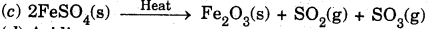(d) Acidic
(e) 3rd period, group 16

Question 24.
An element X of group 15 exists as diatomic molecule and combines with hydrogen at 773 K in presence of the catalyst to form a compound, ammonia which has a characteristic pungent smell.
(a) Identify the element X. How many valence electrons does it have?
(b) Draw-the electron dot structure of the diatomic molecule of X. What type of bond is formed in it?
(c) Draw the electron dot structure for ammonia and what type of bond is formed in it?
(a) Nitrogen (atomic no. 7)Question 25.
Which group of elements could be placed in Mendeléev’g Periodic Table without disturbing the original order? Give reason.